| my account | login-logout | resources | support | catalog | home | get webcard |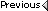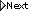» Online Classroom   » Celestial Navigation   » Public Discussion of Cel Nav   » Great Circle Nav by NAO tables

Author Topic: Great Circle Nav by NAO tables
 SRF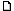posted December 27, 2022 05:11 PM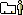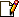Steve Frazer here.I am having trouble getting the correct azimuth when practicing Great Circle Navigation using the NAO tables. If anyone has tried this with the NAO tables I would appreciate hearing back. The correct azimuth is closer to the sum of Z1 + Z2, but following instructions for the NAO tables they are winding up with opposite signs. Or (more likely) I am making a mistake. Start point DR N39-42 x W105-06, destination N51-30 x W00-06.Thanks, Steve
 David Burchposted December 27, 2022 08:24 PMHi Steve, nice to hear from former cel nav students. Glad to help.Let's first check the right answer using starpath.com/calc.39 42N, 105 06W to 51 30N, 00 06W is a GC distance of 4,075.1 nmi at an initial heading of 040.5 This is a spherical earth great circle distance, which is what any cel nav solution should get.For a Cel Nav solution, which is the basic one we need to know, we have to equate the start point with an assumed position, in this case A-lat = 39 42N and a-lon = 105 06 W. For some starting points (in East Lon) this could be tricker, but not this one. Next we have to convert the destination Lat, Lon into a declination, LHA. The declination is N 51 30 and the LHA is how far west of you is the destination, namely 360 00 - 105 06 + 00 06 = 254 60 = 255 00So we need to know Hc and Zn when a-Lat =39 42N, Dec = N 51 30 and LHA = 255 00. We can also check this with a computation at starpath.com/calc before we tackle it with the NAO tables.. We get Hc = 22º 4.9 and Zn 040.5. The initial heading is right, now check the zenith distance 90 - Hc = 89 60 - 22 4.9 = 67º 55.1, which we convert to nmi along a great circle as 67 X 60 + 55.1 = 4020 + 55.1 = 4075.1 nmi, which agrees within rounding errors with the right answer. In short, we can solve great circle computations with sight reduction.Now, finally, we get to the question at hand: Do this sight reduction with the NAO Tables. The easiest solution is use our Starpath work forms and instructions for the process and then check that you got the right answer with Celestial Tools.(Note a subtlety here: I recall you were originally doing these computations with Pub 208, which allows for sight reduction from a DR position of deg and min, whereas the NAO tables, like pub 249, only allows for SR from whole degrees of Lat, so we will have to address that. These tables will take an extra step.)Referring to the figure, with a-Lat = 40 we get Hc = 22º 18 and Zn = 040.8a-Lat = 39 we get Hc = 21º 33 and Zn = 039.9Thus we need to interpolate between 21 78 and 21 33, delta = 45 or 45 x (42/60) = 31.5 up from the lat 39 value or 33+31.5 = 1º 4.5 so Hc at lat 39 42 = 22º 4.5. The interpolated Zn = 42/60 x (40.8-39.9) = 0.6, so final Zn = 39.9 +0.6 = 040.5The exact values are 22º 4.9 and Zn = 040.5And from NAO we get 22º 4.5 and Zn = 040.5If the values in this image do not help find the errors, then let me know and we will fill out our NAO form. The form is online, the instructions are in our cel nav text, and Celestial Tools is in our downloads section.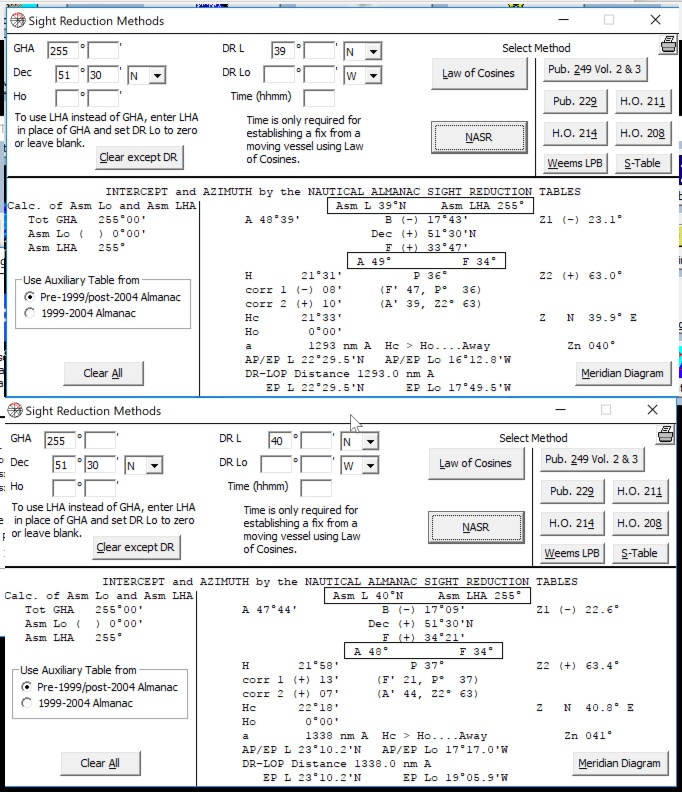From: Starpath, Seattle, WA

 All times are Pacific